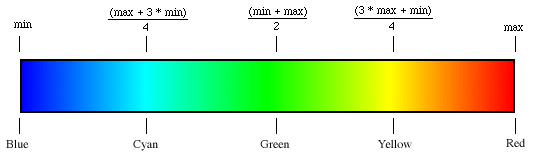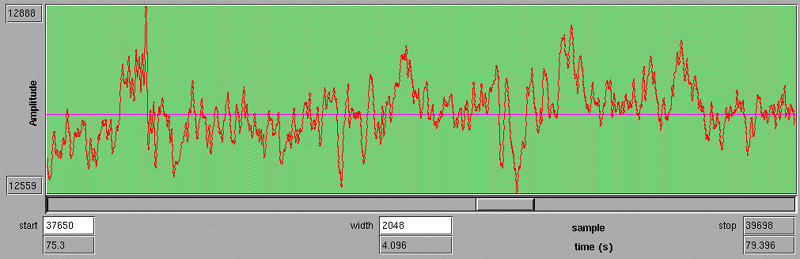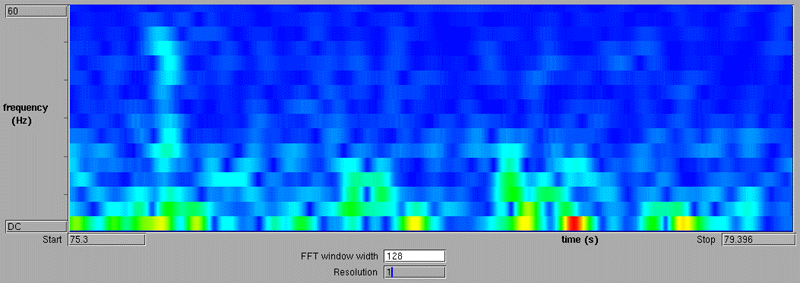# Sonograms

Written by Paul Bourke
January 1998

A sonogram is a means of displaying both time and frequency information at once, as opposed to a single FFT which displays the frequency spectrum of a whole time series. Sonograms have found applications in many areas but are particularly useful in the analysis of speech where the changing frequency content of the signal is of primary interest.

Calculating a sonogram involves computing many short FFT's over the time interval of interest. In order to create smooth transitions in time and form an image of reasonable width the short FFT segments overlap. Typically the overlap is at least 50% and often up to 90%. The magnitude or power of each of these FFT's are "stacked" vertically generating a 2D display, ie: an image.The magnitude of each Fourier component is mapped onto a colour ramp, usually the blue through green to red ramp is used where blue represents low values and red represents high values.There is a trade-off between time and frequency resolution, as the FFT window width is decreased the time resolution increases but the frequency resolution decreases and visa versa (increasing the frequency resolution by increasing the FFT window width decreases the time resolution).

### Example

The following shows a time series segment of 2048 points, a recording from human EEG.The following is the sonogram for the above time series. The discrete nature along the frequency axis is controlled by the width of the FFT window, in this case it is 128 samples, since the sampling frequency is 500Hz this results in a frequency resolution of about 3.9Hz. In addition, the high DC component (signal mean) is removed for scaling purposes.A more unusual method of displaying the sonogram is as a 3D surface with the magnitude (or power) of the frequency components proportional to the height of the surface. The 3D representation of the above sonogram is illustrated below.This does not contain any more information than the more conventional 2D sonogram but the structure is generally easier to appreciate.